# cs231n 2019. A1, part 2. Multiclass Support Vector Machine exercise#### Solution by Yury Kashnitsky (@yorko)

Complete and hand in this completed worksheet (including its outputs and any supporting code outside of the worksheet) with your assignment submission. For more details see the assignments page on the course website.

In this exercise you will:

• implement a fully-vectorized loss function for the SVM
• implement the fully-vectorized expression for its analytic gradient
• use a validation set to tune the learning rate and regularization strength
• optimize the loss function with SGD
• visualize the final learned weights
In :
# Run some setup code for this notebook.

import random
import numpy as np
from tqdm import tqdm_notebook
import matplotlib.pyplot as plt

# This is a bit of magic to make matplotlib figures appear inline in the
# notebook rather than in a new window.
%matplotlib inline
plt.rcParams['figure.figsize'] = (10.0, 8.0) # set default size of plots
plt.rcParams['image.interpolation'] = 'nearest'
plt.rcParams['image.cmap'] = 'gray'

# Some more magic so that the notebook will reload external python modules;


In :
# Load the raw CIFAR-10 data.
cifar10_dir = '/home/yorko/data/cifar-10-batches-py/'
X_train, y_train, X_test, y_test = load_CIFAR10(cifar10_dir)

# As a sanity check, we print out the size of the training and test data.
print('Training data shape: ', X_train.shape)
print('Training labels shape: ', y_train.shape)
print('Test data shape: ', X_test.shape)
print('Test labels shape: ', y_test.shape)

Training data shape:  (50000, 32, 32, 3)
Training labels shape:  (50000,)
Test data shape:  (10000, 32, 32, 3)
Test labels shape:  (10000,)

In :
# Visualize some examples from the dataset.
# We show a few examples of training images from each class.
classes = ['plane', 'car', 'bird', 'cat', 'deer', 'dog', 'frog', 'horse', 'ship', 'truck']
num_classes = len(classes)
samples_per_class = 7
for y, cls in enumerate(classes):
idxs = np.flatnonzero(y_train == y)
idxs = np.random.choice(idxs, samples_per_class, replace=False)
for i, idx in enumerate(idxs):
plt_idx = i * num_classes + y + 1
plt.subplot(samples_per_class, num_classes, plt_idx)
plt.imshow(X_train[idx].astype('uint8'))
plt.axis('off')
if i == 0:
plt.title(cls)
plt.show()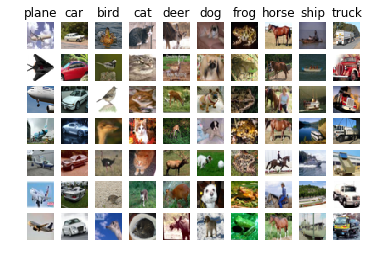In :
# Split the data into train, val, and test sets. In addition we will
# create a small development set as a subset of the training data;
# we can use this for development so our code runs faster.
num_training = 49000
num_validation = 1000
num_test = 1000
num_dev = 500

# Our validation set will be num_validation points from the original
# training set.
mask = range(num_training, num_training + num_validation)

# Our training set will be the first num_train points from the original
# training set.

# We will also make a development set, which is a small subset of
# the training set.

# We use the first num_test points of the original test set as our
# test set.

print('Train data shape: ', X_train.shape)
print('Train labels shape: ', y_train.shape)
print('Validation data shape: ', X_val.shape)
print('Validation labels shape: ', y_val.shape)
print('Test data shape: ', X_test.shape)
print('Test labels shape: ', y_test.shape)

Train data shape:  (49000, 32, 32, 3)
Train labels shape:  (49000,)
Validation data shape:  (1000, 32, 32, 3)
Validation labels shape:  (1000,)
Test data shape:  (1000, 32, 32, 3)
Test labels shape:  (1000,)

In :
# Preprocessing: reshape the image data into rows
X_train = np.reshape(X_train, (X_train.shape, -1))
X_val = np.reshape(X_val, (X_val.shape, -1))
X_test = np.reshape(X_test, (X_test.shape, -1))
X_dev = np.reshape(X_dev, (X_dev.shape, -1))

# As a sanity check, print out the shapes of the data
print('Training data shape: ', X_train.shape)
print('Validation data shape: ', X_val.shape)
print('Test data shape: ', X_test.shape)
print('dev data shape: ', X_dev.shape)

Training data shape:  (49000, 3072)
Validation data shape:  (1000, 3072)
Test data shape:  (1000, 3072)
dev data shape:  (500, 3072)

In :
# Preprocessing: subtract the mean image
# first: compute the image mean based on the training data
mean_image = np.mean(X_train, axis=0)
print(mean_image[:10]) # print a few of the elements
plt.figure(figsize=(4,4))
plt.imshow(mean_image.reshape((32,32,3)).astype('uint8')) # visualize the mean image
plt.show()

[130.64189796 135.98173469 132.47391837 130.05569388 135.34804082
131.75402041 130.96055102 136.14328571 132.47636735 131.48467347]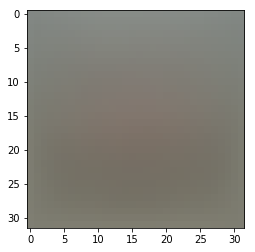In :
# second: subtract the mean image from train and test data
X_train -= mean_image
X_val -= mean_image
X_test -= mean_image
X_dev -= mean_image

In :
# third: append the bias dimension of ones (i.e. bias trick) so that our SVM
# only has to worry about optimizing a single weight matrix W.
X_train = np.hstack([X_train, np.ones((X_train.shape, 1))])
X_val = np.hstack([X_val, np.ones((X_val.shape, 1))])
X_test = np.hstack([X_test, np.ones((X_test.shape, 1))])
X_dev = np.hstack([X_dev, np.ones((X_dev.shape, 1))])

print(X_train.shape, X_val.shape, X_test.shape, X_dev.shape)

(49000, 3073) (1000, 3073) (1000, 3073) (500, 3073)


## SVM Classifier¶

Your code for this section will all be written inside cs231n/classifiers/linear_svm.py.

As you can see, we have prefilled the function compute_loss_naive which uses for loops to evaluate the multiclass SVM loss function.

In :
# Evaluate the naive implementation of the loss we provided for you:
from cs231n.classifiers.linear_svm import svm_loss_naive
import time

# generate a random SVM weight matrix of small numbers
W = np.random.randn(3073, 10) * 0.0001

loss, grad = svm_loss_naive(W, X_dev, y_dev, 0.000005)
print('loss: %f' % (loss, ))

loss: 8.732337


The grad returned from the function above is right now all zero. Derive and implement the gradient for the SVM cost function and implement it inline inside the function svm_loss_naive. You will find it helpful to interleave your new code inside the existing function.

To check that you have correctly implemented the gradient correctly, you can numerically estimate the gradient of the loss function and compare the numeric estimate to the gradient that you computed. We have provided code that does this for you:

In :
# Once you've implemented the gradient, recompute it with the code below
# and gradient check it with the function we provided for you

# Compute the loss and its gradient at W.
loss, grad = svm_loss_naive(W, X_dev, y_dev, 0.0)

# Numerically compute the gradient along several randomly chosen dimensions, and
# compare them with your analytically computed gradient. The numbers should match
# almost exactly along all dimensions.
f = lambda w: svm_loss_naive(w, X_dev, y_dev, 0.0)

# do the gradient check once again with regularization turned on
# you didn't forget the regularization gradient did you?
loss, grad = svm_loss_naive(W, X_dev, y_dev, 5e1)
f = lambda w: svm_loss_naive(w, X_dev, y_dev, 5e1)

numerical: -27.528343 analytic: -27.528343, relative error: 4.356821e-12
numerical: -8.751809 analytic: -8.751809, relative error: 5.264237e-12
numerical: 14.104662 analytic: 14.104662, relative error: 2.962198e-12
numerical: -42.872190 analytic: -42.872190, relative error: 4.082304e-12
numerical: -7.907090 analytic: -7.907090, relative error: 2.805998e-11
numerical: 10.012486 analytic: 10.012486, relative error: 1.185295e-11
numerical: 5.062722 analytic: 5.062722, relative error: 3.885270e-12
numerical: -3.408451 analytic: -3.408451, relative error: 3.854381e-11
numerical: 11.896387 analytic: 11.896387, relative error: 4.089475e-12
numerical: -13.363754 analytic: -13.363754, relative error: 4.074384e-11
numerical: 9.944361 analytic: 9.944361, relative error: 1.950295e-11
numerical: -28.174528 analytic: -28.174528, relative error: 8.574572e-12
numerical: 4.502631 analytic: 4.502631, relative error: 5.843275e-11
numerical: 20.102808 analytic: 20.102808, relative error: 1.067070e-11
numerical: 19.416356 analytic: 19.416356, relative error: 2.658631e-12
numerical: 7.035180 analytic: 7.035180, relative error: 1.685205e-11
numerical: -12.131133 analytic: -12.131133, relative error: 3.127531e-11
numerical: -22.088578 analytic: -22.088578, relative error: 3.021264e-11
numerical: -5.935674 analytic: -5.935674, relative error: 3.238932e-11
numerical: 14.752980 analytic: 14.752980, relative error: 5.203795e-12


### Inline Question #1¶

It is possible that once in a while a dimension in the gradcheck will not match exactly. What could such a discrepancy be caused by? Is it a reason for concern? What is a simple example in one dimension where a gradient check could fail? Hint: the SVM loss function is not strictly speaking differentiable

$\color{blue}{\textit Your Answer:}$ The problem with gradient checking occurs when the margin is strictly equal to zero or is close to zero (is less than step h in gradient checking formula). For 2-class problem this is the case when the difference between scores for the first and second classes is equal to one. This is actually not a big deal, the weights can still be updated in such case.

In :
# Next implement the function svm_loss_vectorized; for now only compute the loss;
# we will implement the gradient in a moment.
tic = time.time()
loss_naive, grad_naive = svm_loss_naive(W, X_dev, y_dev, 0.000005)
toc = time.time()
print('Naive loss: %e computed in %fs' % (loss_naive, toc - tic))

from cs231n.classifiers.linear_svm import svm_loss_vectorized
tic = time.time()
loss_vectorized, _ = svm_loss_vectorized(W, X_dev, y_dev, 0.000005)
toc = time.time()
print('Vectorized loss: %e computed in %fs' % (loss_vectorized, toc - tic))

# The losses should match but your vectorized implementation should be much faster.
print('difference: %f' % (loss_naive - loss_vectorized))

Naive loss: 8.732337e+00 computed in 0.065431s
Vectorized loss: 8.732337e+00 computed in 0.002717s
difference: -0.000000

In :
# Complete the implementation of svm_loss_vectorized, and compute the gradient
# of the loss function in a vectorized way.

# The naive implementation and the vectorized implementation should match, but
# the vectorized version should still be much faster.
tic = time.time()
_, grad_naive = svm_loss_naive(W, X_dev, y_dev, 0.000005)
toc = time.time()
print('Naive loss and gradient: computed in %fs' % (toc - tic))

tic = time.time()
_, grad_vectorized = svm_loss_vectorized(W, X_dev, y_dev, 0.000005)
toc = time.time()
print('Vectorized loss and gradient: computed in %fs' % (toc - tic))

# The loss is a single number, so it is easy to compare the values computed
# by the two implementations. The gradient on the other hand is a matrix, so
# we use the Frobenius norm to compare them.
print('difference: %f' % difference)

Naive loss and gradient: computed in 0.067662s
Vectorized loss and gradient: computed in 0.004179s
difference: 0.000000


We now have vectorized and efficient expressions for the loss, the gradient and our gradient matches the numerical gradient. We are therefore ready to do SGD to minimize the loss.

In :
# In the file linear_classifier.py, implement SGD in the function
# LinearClassifier.train() and then run it with the code below.
from cs231n.classifiers import LinearSVM
svm = LinearSVM()
tic = time.time()
loss_hist = svm.train(X_train, y_train, learning_rate=1e-7, reg=2.5e4,
num_iters=1500, verbose=True)
toc = time.time()
print('That took %fs' % (toc - tic))

iteration 0 / 1500: loss 785.125888
iteration 100 / 1500: loss 288.059997
iteration 200 / 1500: loss 107.500954
iteration 300 / 1500: loss 42.198001
iteration 400 / 1500: loss 18.993530
iteration 500 / 1500: loss 10.579750
iteration 600 / 1500: loss 6.945477
iteration 700 / 1500: loss 6.301501
iteration 800 / 1500: loss 6.195336
iteration 900 / 1500: loss 5.388847
iteration 1000 / 1500: loss 4.822270
iteration 1100 / 1500: loss 5.447574
iteration 1200 / 1500: loss 5.634973
iteration 1300 / 1500: loss 5.265655
iteration 1400 / 1500: loss 5.397747
That took 10.796080s

In :
# A useful debugging strategy is to plot the loss as a function of
# iteration number:
plt.plot(loss_hist)
plt.xlabel('Iteration number')
plt.ylabel('Loss value')
plt.show()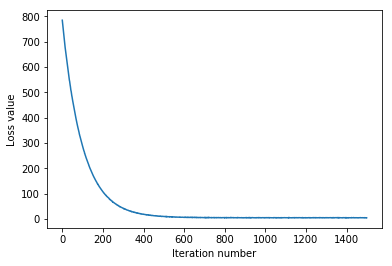In :
# Write the LinearSVM.predict function and evaluate the performance on both the
# training and validation set
y_train_pred = svm.predict(X_train)
print('training accuracy: %f' % (np.mean(y_train == y_train_pred), ))
y_val_pred = svm.predict(X_val)
print('validation accuracy: %f' % (np.mean(y_val == y_val_pred), ))

training accuracy: 0.371735
validation accuracy: 0.382000

In :
%%time
# Use the validation set to tune hyperparameters (regularization strength and
# learning rate). You should experiment with different ranges for the learning
# rates and regularization strengths; if you are careful you should be able to
# get a classification accuracy of about 0.4 on the validation set.
learning_rates = np.linspace(1.5e-7, 3e-7, 10)
regularization_strengths = np.linspace(0.5e3, 2e4, 10)

# results is dictionary mapping tuples of the form
# (learning_rate, regularization_strength) to tuples of the form
# (training_accuracy, validation_accuracy). The accuracy is simply the fraction
# of data points that are correctly classified.
results = {}
best_val = -1   # The highest validation accuracy that we have seen so far.
best_svm = None # The LinearSVM object that achieved the highest validation rate.

################################################################################
# TODO:                                                                        #
# Write code that chooses the best hyperparameters by tuning on the validation #
# set. For each combination of hyperparameters, train a linear SVM on the      #
# training set, compute its accuracy on the training and validation sets, and  #
# store these numbers in the results dictionary. In addition, store the best   #
# validation accuracy in best_val and the LinearSVM object that achieves this  #
# accuracy in best_svm.                                                        #
#                                                                              #
# Hint: You should use a small value for num_iters as you develop your         #
# validation code so that the SVMs don't take much time to train; once you are #
# confident that your validation code works, you should rerun the validation   #
# code with a larger value for num_iters.                                      #
################################################################################
for lr in tqdm_notebook(learning_rates):
for reg in tqdm_notebook(regularization_strengths):
svm = LinearSVM()
_ = svm.train(X_train, y_train, learning_rate=lr,
reg=reg,
num_iters=1500, verbose=False)
y_train_pred = svm.predict(X_train)
train_acc = np.mean(y_train == y_train_pred)
y_val_pred = svm.predict(X_val)
val_acc = np.mean(y_val == y_val_pred)
results[(lr, reg)] = (train_acc, val_acc)
if val_acc > best_val:
best_val = val_acc
best_svm = svm
################################################################################
#                              END OF YOUR CODE                                #
################################################################################

# Print out results.
for lr, reg in sorted(results):
train_accuracy, val_accuracy = results[(lr, reg)]
print('lr %e reg %e train accuracy: %f val accuracy: %f' % (
lr, reg, train_accuracy, val_accuracy))

print('best validation accuracy achieved during cross-validation: %f' % best_val)

lr 1.500000e-07 reg 5.000000e+02 train accuracy: 0.325592 val accuracy: 0.360000
lr 1.500000e-07 reg 2.666667e+03 train accuracy: 0.375796 val accuracy: 0.370000
lr 1.500000e-07 reg 4.833333e+03 train accuracy: 0.386796 val accuracy: 0.378000
lr 1.500000e-07 reg 7.000000e+03 train accuracy: 0.388306 val accuracy: 0.391000
lr 1.500000e-07 reg 9.166667e+03 train accuracy: 0.383041 val accuracy: 0.384000
lr 1.500000e-07 reg 1.133333e+04 train accuracy: 0.379531 val accuracy: 0.392000
lr 1.500000e-07 reg 1.350000e+04 train accuracy: 0.368163 val accuracy: 0.382000
lr 1.500000e-07 reg 1.566667e+04 train accuracy: 0.377041 val accuracy: 0.381000
lr 1.500000e-07 reg 1.783333e+04 train accuracy: 0.374633 val accuracy: 0.371000
lr 1.500000e-07 reg 2.000000e+04 train accuracy: 0.366959 val accuracy: 0.369000
lr 1.666667e-07 reg 5.000000e+02 train accuracy: 0.332653 val accuracy: 0.355000
lr 1.666667e-07 reg 2.666667e+03 train accuracy: 0.381245 val accuracy: 0.388000
lr 1.666667e-07 reg 4.833333e+03 train accuracy: 0.383816 val accuracy: 0.388000
lr 1.666667e-07 reg 7.000000e+03 train accuracy: 0.381163 val accuracy: 0.370000
lr 1.666667e-07 reg 9.166667e+03 train accuracy: 0.373653 val accuracy: 0.371000
lr 1.666667e-07 reg 1.133333e+04 train accuracy: 0.374122 val accuracy: 0.378000
lr 1.666667e-07 reg 1.350000e+04 train accuracy: 0.367633 val accuracy: 0.377000
lr 1.666667e-07 reg 1.566667e+04 train accuracy: 0.370776 val accuracy: 0.378000
lr 1.666667e-07 reg 1.783333e+04 train accuracy: 0.364306 val accuracy: 0.367000
lr 1.666667e-07 reg 2.000000e+04 train accuracy: 0.369959 val accuracy: 0.383000
lr 1.833333e-07 reg 5.000000e+02 train accuracy: 0.342184 val accuracy: 0.351000
lr 1.833333e-07 reg 2.666667e+03 train accuracy: 0.385939 val accuracy: 0.381000
lr 1.833333e-07 reg 4.833333e+03 train accuracy: 0.389347 val accuracy: 0.397000
lr 1.833333e-07 reg 7.000000e+03 train accuracy: 0.384327 val accuracy: 0.379000
lr 1.833333e-07 reg 9.166667e+03 train accuracy: 0.382571 val accuracy: 0.380000
lr 1.833333e-07 reg 1.133333e+04 train accuracy: 0.373408 val accuracy: 0.390000
lr 1.833333e-07 reg 1.350000e+04 train accuracy: 0.372449 val accuracy: 0.390000
lr 1.833333e-07 reg 1.566667e+04 train accuracy: 0.372510 val accuracy: 0.370000
lr 1.833333e-07 reg 1.783333e+04 train accuracy: 0.374694 val accuracy: 0.368000
lr 1.833333e-07 reg 2.000000e+04 train accuracy: 0.367878 val accuracy: 0.374000
lr 2.000000e-07 reg 5.000000e+02 train accuracy: 0.341347 val accuracy: 0.344000
lr 2.000000e-07 reg 2.666667e+03 train accuracy: 0.384204 val accuracy: 0.379000
lr 2.000000e-07 reg 4.833333e+03 train accuracy: 0.382061 val accuracy: 0.396000
lr 2.000000e-07 reg 7.000000e+03 train accuracy: 0.380367 val accuracy: 0.396000
lr 2.000000e-07 reg 9.166667e+03 train accuracy: 0.378898 val accuracy: 0.383000
lr 2.000000e-07 reg 1.133333e+04 train accuracy: 0.370531 val accuracy: 0.383000
lr 2.000000e-07 reg 1.350000e+04 train accuracy: 0.371163 val accuracy: 0.389000
lr 2.000000e-07 reg 1.566667e+04 train accuracy: 0.372776 val accuracy: 0.376000
lr 2.000000e-07 reg 1.783333e+04 train accuracy: 0.369571 val accuracy: 0.377000
lr 2.000000e-07 reg 2.000000e+04 train accuracy: 0.355245 val accuracy: 0.365000
lr 2.166667e-07 reg 5.000000e+02 train accuracy: 0.340980 val accuracy: 0.343000
lr 2.166667e-07 reg 2.666667e+03 train accuracy: 0.387122 val accuracy: 0.391000
lr 2.166667e-07 reg 4.833333e+03 train accuracy: 0.386980 val accuracy: 0.387000
lr 2.166667e-07 reg 7.000000e+03 train accuracy: 0.388959 val accuracy: 0.381000
lr 2.166667e-07 reg 9.166667e+03 train accuracy: 0.387061 val accuracy: 0.389000
lr 2.166667e-07 reg 1.133333e+04 train accuracy: 0.374633 val accuracy: 0.363000
lr 2.166667e-07 reg 1.350000e+04 train accuracy: 0.368735 val accuracy: 0.378000
lr 2.166667e-07 reg 1.566667e+04 train accuracy: 0.363327 val accuracy: 0.365000
lr 2.166667e-07 reg 1.783333e+04 train accuracy: 0.369490 val accuracy: 0.387000
lr 2.166667e-07 reg 2.000000e+04 train accuracy: 0.363633 val accuracy: 0.375000
lr 2.333333e-07 reg 5.000000e+02 train accuracy: 0.348510 val accuracy: 0.349000
lr 2.333333e-07 reg 2.666667e+03 train accuracy: 0.389061 val accuracy: 0.393000
lr 2.333333e-07 reg 4.833333e+03 train accuracy: 0.390265 val accuracy: 0.407000
lr 2.333333e-07 reg 7.000000e+03 train accuracy: 0.382878 val accuracy: 0.379000
lr 2.333333e-07 reg 9.166667e+03 train accuracy: 0.379000 val accuracy: 0.381000
lr 2.333333e-07 reg 1.133333e+04 train accuracy: 0.374408 val accuracy: 0.384000
lr 2.333333e-07 reg 1.350000e+04 train accuracy: 0.370714 val accuracy: 0.381000
lr 2.333333e-07 reg 1.566667e+04 train accuracy: 0.377776 val accuracy: 0.379000
lr 2.333333e-07 reg 1.783333e+04 train accuracy: 0.362469 val accuracy: 0.367000
lr 2.333333e-07 reg 2.000000e+04 train accuracy: 0.366143 val accuracy: 0.377000
lr 2.500000e-07 reg 5.000000e+02 train accuracy: 0.352265 val accuracy: 0.347000
lr 2.500000e-07 reg 2.666667e+03 train accuracy: 0.394571 val accuracy: 0.391000
lr 2.500000e-07 reg 4.833333e+03 train accuracy: 0.385898 val accuracy: 0.393000
lr 2.500000e-07 reg 7.000000e+03 train accuracy: 0.381490 val accuracy: 0.368000
lr 2.500000e-07 reg 9.166667e+03 train accuracy: 0.373429 val accuracy: 0.384000
lr 2.500000e-07 reg 1.133333e+04 train accuracy: 0.364939 val accuracy: 0.386000
lr 2.500000e-07 reg 1.350000e+04 train accuracy: 0.365857 val accuracy: 0.393000
lr 2.500000e-07 reg 1.566667e+04 train accuracy: 0.366020 val accuracy: 0.369000
lr 2.500000e-07 reg 1.783333e+04 train accuracy: 0.361082 val accuracy: 0.380000
lr 2.500000e-07 reg 2.000000e+04 train accuracy: 0.360939 val accuracy: 0.367000
lr 2.666667e-07 reg 5.000000e+02 train accuracy: 0.356592 val accuracy: 0.339000
lr 2.666667e-07 reg 2.666667e+03 train accuracy: 0.393939 val accuracy: 0.373000
lr 2.666667e-07 reg 4.833333e+03 train accuracy: 0.387755 val accuracy: 0.371000
lr 2.666667e-07 reg 7.000000e+03 train accuracy: 0.376449 val accuracy: 0.375000
lr 2.666667e-07 reg 9.166667e+03 train accuracy: 0.374122 val accuracy: 0.369000
lr 2.666667e-07 reg 1.133333e+04 train accuracy: 0.366102 val accuracy: 0.380000
lr 2.666667e-07 reg 1.350000e+04 train accuracy: 0.362245 val accuracy: 0.371000
lr 2.666667e-07 reg 1.566667e+04 train accuracy: 0.363408 val accuracy: 0.384000
lr 2.666667e-07 reg 1.783333e+04 train accuracy: 0.352469 val accuracy: 0.364000
lr 2.666667e-07 reg 2.000000e+04 train accuracy: 0.364408 val accuracy: 0.362000
lr 2.833333e-07 reg 5.000000e+02 train accuracy: 0.361286 val accuracy: 0.339000
lr 2.833333e-07 reg 2.666667e+03 train accuracy: 0.392143 val accuracy: 0.399000
lr 2.833333e-07 reg 4.833333e+03 train accuracy: 0.386694 val accuracy: 0.381000
lr 2.833333e-07 reg 7.000000e+03 train accuracy: 0.377633 val accuracy: 0.380000
lr 2.833333e-07 reg 9.166667e+03 train accuracy: 0.372041 val accuracy: 0.377000
lr 2.833333e-07 reg 1.133333e+04 train accuracy: 0.353980 val accuracy: 0.358000
lr 2.833333e-07 reg 1.350000e+04 train accuracy: 0.371510 val accuracy: 0.387000
lr 2.833333e-07 reg 1.566667e+04 train accuracy: 0.347735 val accuracy: 0.342000
lr 2.833333e-07 reg 1.783333e+04 train accuracy: 0.346082 val accuracy: 0.363000
lr 2.833333e-07 reg 2.000000e+04 train accuracy: 0.361653 val accuracy: 0.354000
lr 3.000000e-07 reg 5.000000e+02 train accuracy: 0.358306 val accuracy: 0.365000
lr 3.000000e-07 reg 2.666667e+03 train accuracy: 0.377571 val accuracy: 0.377000
lr 3.000000e-07 reg 4.833333e+03 train accuracy: 0.377918 val accuracy: 0.377000
lr 3.000000e-07 reg 7.000000e+03 train accuracy: 0.374306 val accuracy: 0.370000
lr 3.000000e-07 reg 9.166667e+03 train accuracy: 0.366327 val accuracy: 0.371000
lr 3.000000e-07 reg 1.133333e+04 train accuracy: 0.351429 val accuracy: 0.358000
lr 3.000000e-07 reg 1.350000e+04 train accuracy: 0.351306 val accuracy: 0.352000
lr 3.000000e-07 reg 1.566667e+04 train accuracy: 0.359245 val accuracy: 0.369000
lr 3.000000e-07 reg 1.783333e+04 train accuracy: 0.356490 val accuracy: 0.363000
lr 3.000000e-07 reg 2.000000e+04 train accuracy: 0.352306 val accuracy: 0.358000
best validation accuracy achieved during cross-validation: 0.407000
CPU times: user 2h 43min 47s, sys: 5.12 s, total: 2h 43min 52s
Wall time: 32min 52s

In :
# Visualize the cross-validation results
import math
x_scatter = [math.log10(x) for x in results]
y_scatter = [math.log10(x) for x in results]

# plot training accuracy
marker_size = 100
colors = [results[x] for x in results]
plt.subplot(2, 1, 1)
plt.scatter(x_scatter, y_scatter, marker_size, c=colors)
plt.colorbar()
plt.xlabel('log learning rate')
plt.ylabel('log regularization strength')
plt.title('CIFAR-10 training accuracy')

# plot validation accuracy
colors = [results[x] for x in results] # default size of markers is 20
plt.subplot(2, 1, 2)
plt.scatter(x_scatter, y_scatter, marker_size, c=colors)
plt.colorbar()
plt.xlabel('log learning rate')
plt.ylabel('log regularization strength')
plt.title('CIFAR-10 validation accuracy')
plt.show()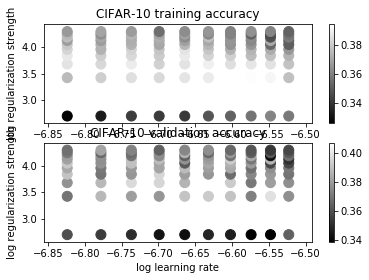In :
y_test_pred = best_svm.predict(X_test)
test_accuracy = np.mean(y_test == y_test_pred)
print('linear SVM on raw pixels final test set accuracy: %f' % test_accuracy)

linear SVM on raw pixels final test set accuracy: 0.375000

In :
# Visualize the learned weights for each class.
# Depending on your choice of learning rate and regularization strength, these may
# or may not be nice to look at.
w = best_svm.W[:-1,:] # strip out the bias
w = w.reshape(32, 32, 3, 10)
w_min, w_max = np.min(w), np.max(w)
classes = ['plane', 'car', 'bird', 'cat', 'deer', 'dog', 'frog', 'horse', 'ship', 'truck']
for i in range(10):
plt.subplot(2, 5, i + 1)

# Rescale the weights to be between 0 and 255
wimg = 255.0 * (w[:, :, :, i].squeeze() - w_min) / (w_max - w_min)
plt.imshow(wimg.astype('uint8'))
plt.axis('off')
plt.title(classes[i])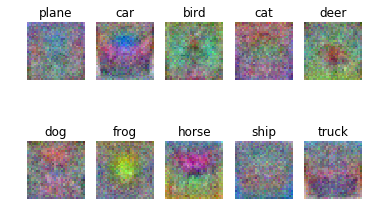### Inline question #2¶

Describe what your visualized SVM weights look like, and offer a brief explanation for why they look the way that they do.

$\color{blue}{\textit Your Answer:}$ The weights serve as a sort of templates for each class, for new image the cross-product between this image pixels and weights (templates) will show how similar these matrices are, i.e. to what extent the image matches the corresponding template. As we can see, if visualized, the weight matrices convey some general features of the representatives of each class.